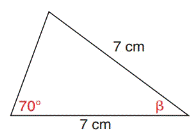Chapter 11.4, Problem 20E### Elementary Geometry for College St...

6th Edition
Daniel C. Alexander + 1 other
ISBN: 9781285195698

#### Solutions

Chapter
Section### Elementary Geometry for College St...

6th Edition
Daniel C. Alexander + 1 other
ISBN: 9781285195698
Textbook Problem
1 views

# In Exercises 17 to 22, use a form of the Law of Sines to find the measure of the indicated side or angle. Angle measures should be found to the nearest degree and lengths of sides to the nearest tenth of a unit.To determine

To find:

The angle of the given triangle by using the Law of Sines.

Explanation

Formula:

The Law of Sines,

sinαa=sinβb=sinγc

Where α, β, and γ is the acute angle of the triangle, and a, b, and c is the sides of the triangle.

Calculation:

Given,

From the figure,

a=3 cm.,

c=7 cm.,

α=70°

Find γ:

By the Law of Sines,

sinαa=sinβb=sinγc

Take,

sinαa=sinγc

Substitute a=7 cm, b=7 cm, and γ=70°

sin70°7=sinγ7

sin70°=sinγ

(i

### Still sussing out bartleby?

Check out a sample textbook solution.

See a sample solution

#### The Solution to Your Study Problems

Bartleby provides explanations to thousands of textbook problems written by our experts, many with advanced degrees!

Get Started

#### Simplify the expressions in Exercises 97106. y5/4y3/4

Finite Mathematics and Applied Calculus (MindTap Course List)

#### In Problems 5-8, use technology to graph the functions. 6.

Mathematical Applications for the Management, Life, and Social Sciences

#### Evaluate the integral. 14. sin2(1/t)t2dt

Single Variable Calculus: Early Transcendentals